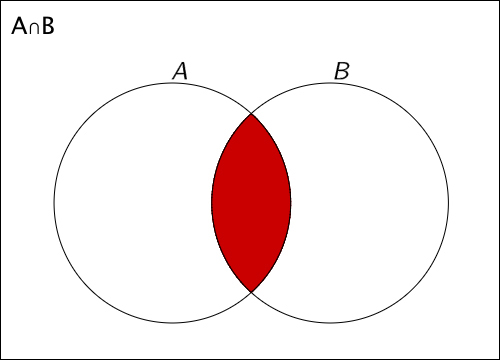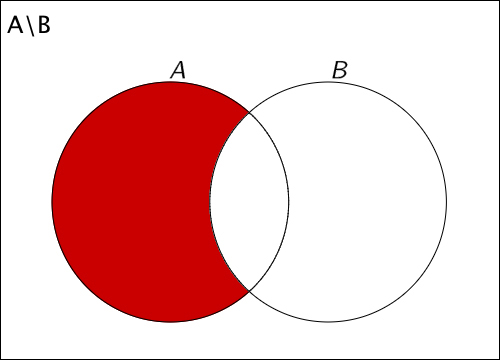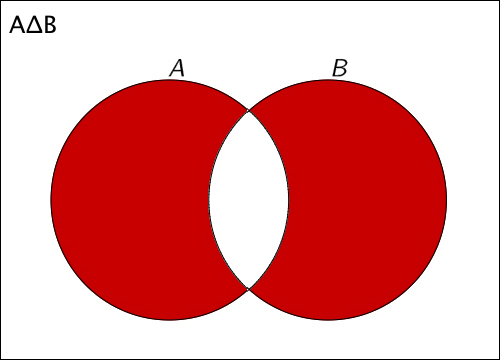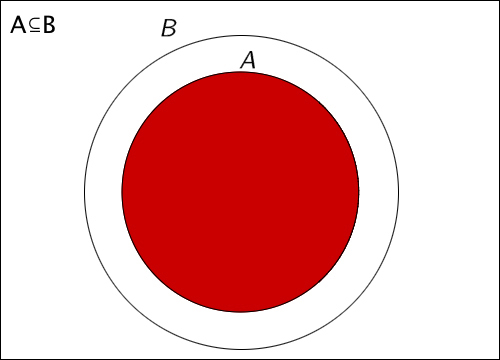# Operations on Sets

Keywords: sets, union, intersection, difference, symmetric difference, subsets, complements

## Union

$A\cup B=\left\{x|x\in A\vee x\in B\right\}$## Intersection

$A\cap B=\left\{x|x\in A\wedge x\in B\right\}$## Difference

$A\setminus B=\left\{x|x\in A\wedge x\notin B\right\}$## Symmetric Difference

$A\Delta B=\left\{A\setminus B\right\}\cup \left\{B\setminus A\right\}$## Subsets

$A\subseteq B$## Complements

$\overline{A}=\left\{x|x\in B\wedge x\notin A\right\}$Halmazok úni